Complex Numbers

 The graphing calculator can be a very useful tool for checking your work with complex numbers. Keep in mind, when working with a graphing calculator, that there may be more than one way to arrive at an answer.

 Use the MODE key to place the calculator in a+bi mode.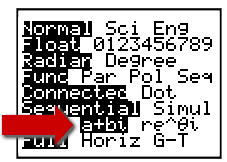The complex i  is found above the decimal point key or in the catalog.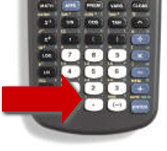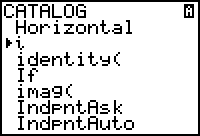Note:  Complex numbers can be accessed from Real Mode (without placing calculator in a + bi Mode).  Real mode, however, does not display complex results unless complex numbers are entered as input.  For example, if the calculator is NOT in a + bi Mode,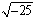will create an error.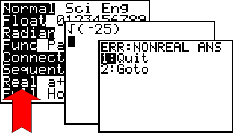You get an error message when you try to enter the square root of a negative number when in Real Mode.You need to be in a + bi mode to evaluate the square root of a negative number.

Now, let's look at the arithmetic of complex numbers:

 Add:  (2 + 4i) + (3 - 2i) Subtract: (6 - 3i) - (4 + 5i) Multiply: (3 + 2i) (4 - 2i)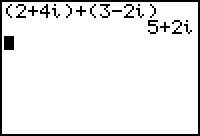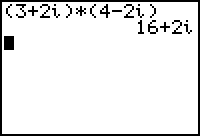Divide :  (2+3i)/(4-3i) (answer appears in a + bi form)   WOW!! The calculator did the conjugate work for you. YEA! For TI-83+ and for TI-84+ with OS prior to OS 2.53MP.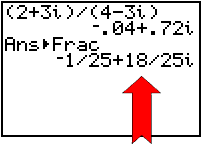In this display, i is NOT in the denominator!  The calculator is simply listing order of operations. TI-84+ with OS 2.53MPTI-84+ CE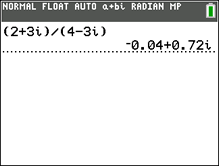As a fraction, asnwer is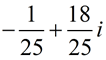Be careful to write your final answer correctly.  Note the location of the i in the final answer:The i can also be written in the numerator with 18, but it cannot be written in the denominator with 25.NOTE: On OS2.53MP or the CE, if the decimals you are trying to "grab" to convert to fractions are really long, use MATH - CPX - real( and imag( to "grab" the full decimal value for converting "a" and "b" to fractions.

Using the calculator to investigate powers of i:

 Investigate the powers of i.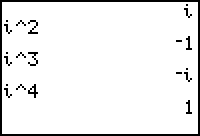These values will appear when you are in either Real Mode or in a + bi mode. You can look at many powers at once by using a list... use right arrow to scroll to the right to see all of the answers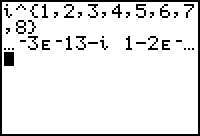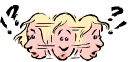What kind of number is - 3E - 13 - i ??? This number is really just - i. - 3E - 13 is so small, it is considered to be zero. (E-13 is Scientific Notation meaning 10 raised to -13 power.) The older the calculator, the "sooner" you will start to see these scientific notation answers. The TI-84+CE is better with finding powers of i, but it, as well, will resort to the slightly less accurate scientific notation listings as the powers get larger. ------> TI-84+CE is OK through a power of 100.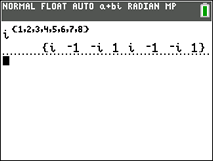"My Deer, BEWARE!!! When raising i to a power on the graphing calculator, accuracy diminishes as the powers increase.

We know mathematically
that i178 is i2 which is -1.

On all of the TI-84+ family calculators,
i178 = -1+2.2E-12i.

But it is actually just -1.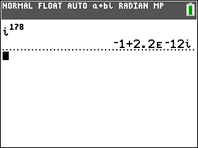Special Calculator Functions for Complex Numbers
:

There are also special functions on the graphing calculator to deal with complex numbers
(but you probably won't need a calculator for many of these functions):

 Hit MATH key and arrow to the right to CPX:1. conj(  returns the complex conjugate of a complex number.     conj(2+5i)  gives  2-5i 2. real(   returns the "a" value in an a+bi complex number.     real(2+5i)  gives  2 3. imag(   returns the "b" value in an a+bi complex number.     imag(2+5i)  gives  5 4. angle(  returns the angle, or argument, of the complex number - the angle formed by the positive x-axis (the positive real axis) and the segment from the origin to the complex number point on an Argand diagram.     angle(2+5i)  gives  68.199º (with calculator set in degree mode) 5.  abs(   returns the absolute value (magnitude) of the complex number.      abs(5+12i)  gives  13      abs (2 + 5i)  gives  5.385164807 (Note:  The absolute value of a complex number may also be called its magnitude.  It you plot a complex number as a single point, the absolute value represents the distance from the origin to that point.  If you plot a complex number as a vector, the absolute value represents the length of the vector.)